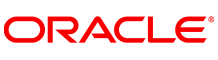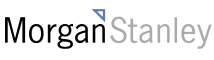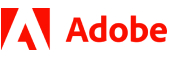New update is available. Click here to update.
Topics

# Find Two Missing Numbers

Moderate0/80
Average time to solve is 25m+3 more companies

## Problem statement

You are given an array of unique integers where each element in the array is in the range [1, N]. The array has all distinct elements, and the array’s size is (N - 2). Hence, two numbers from the range are missing from this array. Your task is to return the two missing numbers.

For Example :

``````If ‘N’ = 6
[1, 3, 6, 5]
Then the output will be 2 4.
``````
Detailed explanation ( Input/output format, Notes, Images )
Sample Input 1 :
``````2
6
1 3 5 6
3
1
``````
Sample Output 1 :
``````2 4
2 3
``````
Explanation of Sample Input 1 :
``````The two elements that are missing in the range [1, 6] are 2 and 4.
The two elements missing in the range [1,3] are 2 and 3.
``````
Sample Input 2 :
``````2
5
1 3 4
7
2 3 4 5 6
``````
Sample Output 2 :
``````2 5
1 7
``````Console